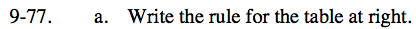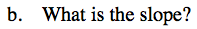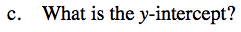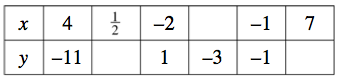9-77.

Missing problem for Book CC3 => Chapter Ch9 => Lesson 9.2.2 => Problem 9-77

Created from orphaned homework help problemSee problem 9-58 for help in finding the rule of the table.

Rule: y = −2x − 3
What would the slope be based on this equation?

It might be easier to use the points (−2, 1) and (−1, −1) to find the slope.

Explore the problem with the eTool below.
Click the link at right for the full version of the eTool: CC3 9-77 HW eTool